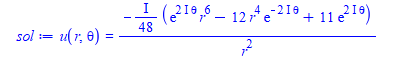# Question:pdsolve problem with Laplace pde on disk using polar coordinates

## Question:pdsolve problem with Laplace pde on disk using polar coordinates

Maple 2021

I can't get pdsolve to solve this pde. Here are my tries below. One of them works, but the analytical solution Maple gives is wrong. So I am not sure if it needs some additional hints or some other help to make it give the correct solution.

This is Laplace pde but with non-zero on RHS. On disk centered at origin and with radius 1, with given BC on edge of disk.

```restart;
the_rhs := r^2*cos(theta)*sin(theta);
pde := VectorCalculus:-Laplacian(u(r,theta),'polar'[r,theta])=the_rhs;
bc := u(1, theta) = cos(theta)*sin(theta);
sol:=pdsolve([pde, bc], u(r, theta));
```

Maple givesBut this does not look right. There is a complex exponential on its own there. The solution should be real.

I tried to solve it using pdsolve numerically, but could not make it work. It kepts complaining about missing BC. I am not good with numerical solvers in Maple. May be someone could try to do it.

`evalf(eval(rhs(sol),[r= sqrt(1/4 + 1/4),theta=Pi/4]))`

gives 0.5951891582 which is wrong,. It should be  0.23958

It tried to give it the symmetry conditions on theta, but now it did not solve it

```restart;
the_rhs := r^2*cos(theta)*sin(theta);
pde := VectorCalculus:-Laplacian(u(r,theta),'polar'[r,theta])=the_rhs;
bc := u(1, theta) = cos(theta)*sin(theta),u(r,0) = u(r,2*Pi),(D(u))(r, 0) = (D(u))(r, 2*Pi);
sol:=pdsolve([pde, bc], u(r, theta));
```

No solution. I tried adding HINT = boundedseries(r=0) but that also did not help. No solution.

Could someone solve this PDE numerically in Maple and check the solution at the above location? It should be 0.23958 which shows the analytical solution given is not correct. Can Maple solve this numerically?

Maple 2021.1

ps. Analytical solution was obtained by Mathematica. I did not solve this by hand.﻿